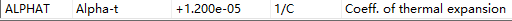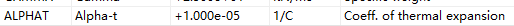# Thermal expansion coefficient (Alpha) doesn't take effect in composite section under uniform Temperature Loading

For composite section, such as steel and concrete section, the steel material is genrally used as the “main” material for composite section, while the concrete is used as “secondary” material.

Under temerature loading, different Alpha values should be used for steel and concrete materials, such as 1.2E-5 for steel and 1.0E-5 for concrete.

In Sofistik 2023-5, a universal Alpha value, 1.2E-5 is used for both steel and concrete under uniform temperature loading, even if I explcitly apply “ALPH 1.0E-5” on the concrete.
This is incorrect.

But under nonlinear temperature loading, I can apply different Alpha values, such as 1.2E-5 for steel and 1.0E-5 for concrete, which is correct.

Below is an example:
If applying 10 degree uniform load on the arch in Sofistik

LC NO TYPE TITL
6 TU 整体升温
LINE REF NO TYPE P1
BGRP (11 61 1) DT 10

This is the moment diagram of the arch

LET#T 10 10 10
LET#Z ‘T1’ ‘T2’ ‘T3’
LOOP#i T
BEAM FROM TO TYPE PA REFT
‘GRP’ 1 T #T(#i) #Z(#i)
ENDLOOP
EVAL TYPE TEIG

I also use RM Bridge (TDV) as comparison

1. Set alpha = 1.2E-5 for concrete, the result is the same as that in Uniform temperature load in Sofistik, which means in Sofistik Uniform temperature Load DT, Sofistik set concrete alpha value the same as that for steel, which is incorrect.2. Set alpha = 1.0E-5, the result is the same as that in Uniform load (by using Nonlinear temperature load) in Sofistik, which means in Sofistik Nonlinear temperature load, Sofistik use the correct alpha value for concrete.Hi, Netsonic!
If composite section will be initially taken as concrete, alfa will be 1e-5, if - as steel, alfa will be 1.2e-5.
In this case, the reduced areas of these sections are not equal to the sum of the areas of concrete and steel, and the modulus of elasticity is taken equal to the value of the modulus of the determining material, as well as the coefficient of thermal expansion. In any case, for composite sections, the coefficient of thermal expansion can be taken in accordance with the note 6 of table С1 EN 1991-1-5:2003 for composite structures the coefficient of linear expansion of the steel component may be taken as equal to 1e-5 to neglect restraining effects from different aT-values.

1 Like

Netsonic, hi!
Yes, really so, you are right.
It is very good way for desired gole.
We can use 2 geometric points (one for concrete, one for steel) and T + EVAL in sofiload.
Best regards

1 Like